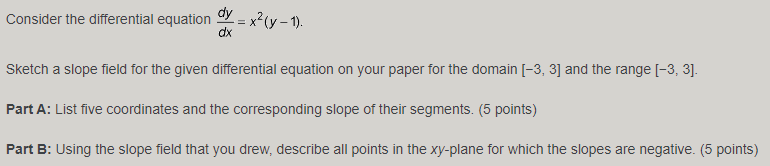# Question Consider the differential equation dy dx = x? (y – 1). Sketch a slope field for the given differential equation on your paper for the domain (-3, 3] and the range (-3, 3). Part A: List five coordinates and the corresponding slope of their segments. (5 points) Part B: Using the slope field that you drew, describe all points in the xy-plane for which the slopes are negative. (5 points)